×

Search anything:

# Supervised, Unsupervised and Semi-Supervised Learning

#### Machine Learning (ML) Deep Learning• Supervised learning
• Unsupervised learning
• Semi-Supervised learning
• Summary

In machine learning, the tasks are broadly categorized into supervised and unsupervised learning. Semi-supervised learning falls between supervised and unsupervised learning. Let us understand what these terms mean.

# Supervised learning

In supervised learning, we have a basic idea beforehand as to what the result is going to be. The input data is labeled and the main task of the model is to either map the output with the input labels or when the input label is mapped as a continuous output. The former method is called classification and the latter is known as th regression. The datasets used are designed to supervise these algorithms into performing their tasks. They have feedback mechanisms which enables this. Hence the name Supervised learning. some examples of supervised learning algorithms are linear regression, logistic regression and Bayes algorithm.

As mentioned above, supervised learning can be separated into two types:

• Classification: These algorithms are used to categorize the given test data accurately, such as telling apart a cat from a dog.Some examples of classification algorithms are linear classifiers, decision trees and k-nearest neighbor.

• Regression: These algorithms are used for finding relationships between the dependent and independent variables. The main goal of a regression model is to come up with an equation for the dependent variable in terms of the given independent variables. This is usually used for predicting and forecasting. Some regression algorithms include linear regression and polynomial regression.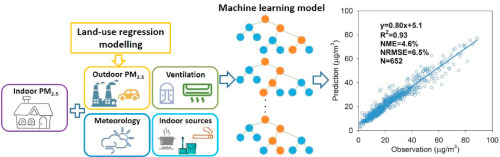## Examples of Supervised learning:

• A real world example of classification includes classifying spam emails apart from others and move them to a separate folder.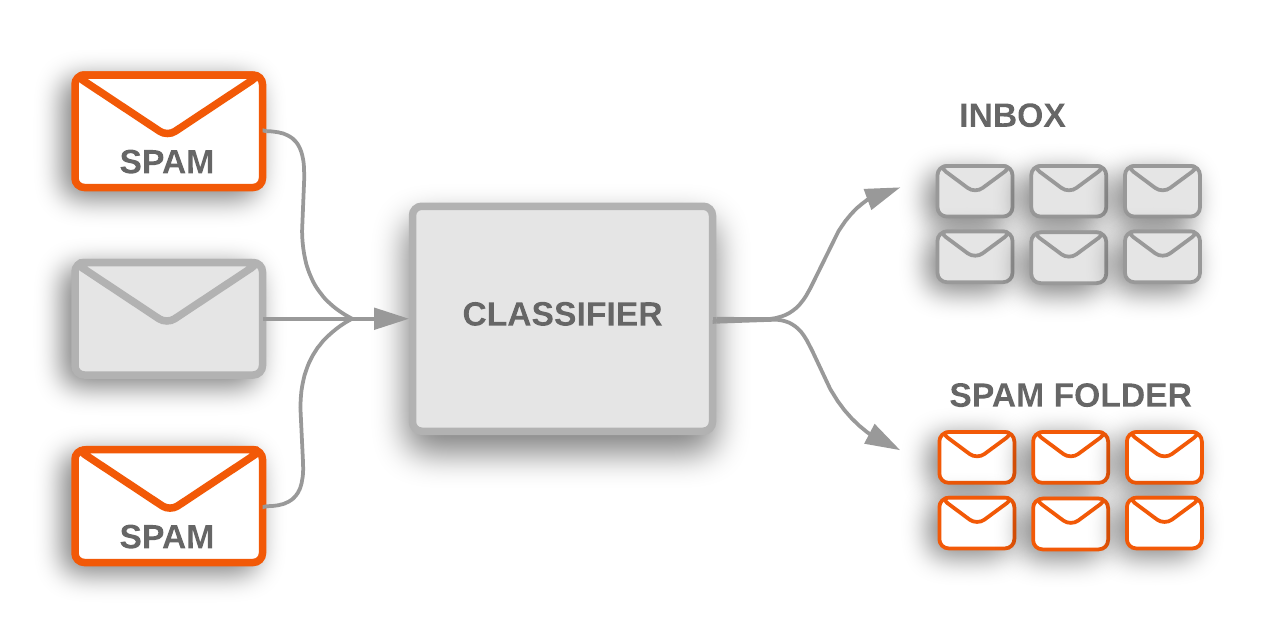• Another use case of classification algorithm is sentiment analysis. It can be used to analyse the feedback given by customers in form of text to find out what opinion the text expresses. Some words in the text like "poor" and "bad" could be classified as a negative review, whereas words like "good" and "great" may indicate a positive review.
• Regression is used to predict and forecast weather conditions. Simply put, the temperature estimates for the next day or week may be predicted using various dependent variables such as humidity, area's elevation, amount of solar energy received and proximity to large water bodies.
• The use of regression analysis in business is for forecasting future opportunities and threats. Demand analysis, as an example, forecasts the number of things a customer is probably going to shop for. The best bid for an advertising can be predicted by forecasting the number of consumers who pass ahead of the particular billboard.
• Classification algorithms are also used in classification and identification of biometrics. The support vector machine algorithm is used to classify and identify fingerprints by analyzing the ridges and curves in the fingerprints.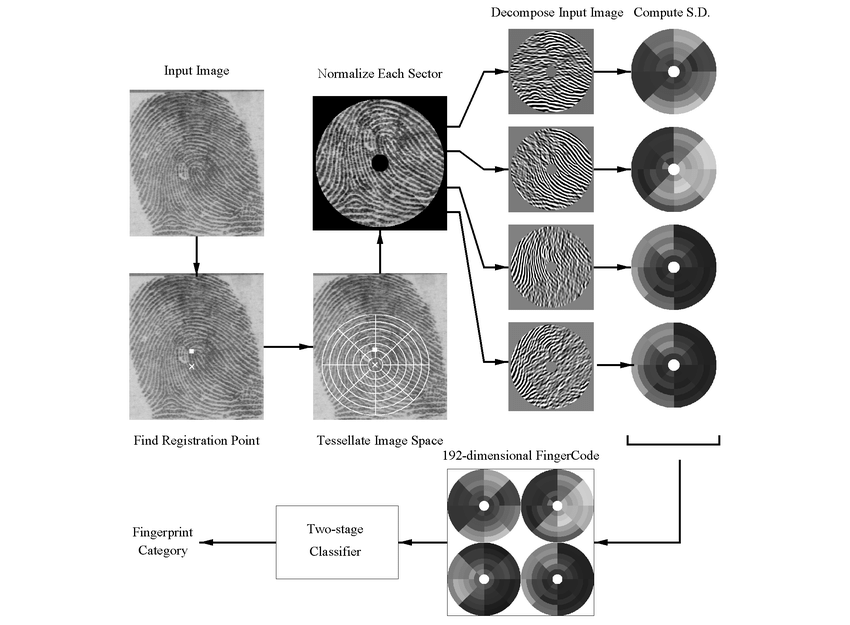# Unsupervised learning

In unsupervised learning, the data is unlabeled and its goal is to find out the natural patterns present within data points in the given dataset. It does not have a feedback mechanism unlike supervised learning and hence this technique is known as unsupervised learning.

The two common uses of unsupervised learning are :

• Clustering: This technique is for grouping the unlabeled data based on their similarities or differences. One of the popular clustering algorithm is K-Means Clustering where it sets aside the data points into different groups and K represents the number of groups. A common example for the use case of K-means clustering is grouping books into different genres based on their summary.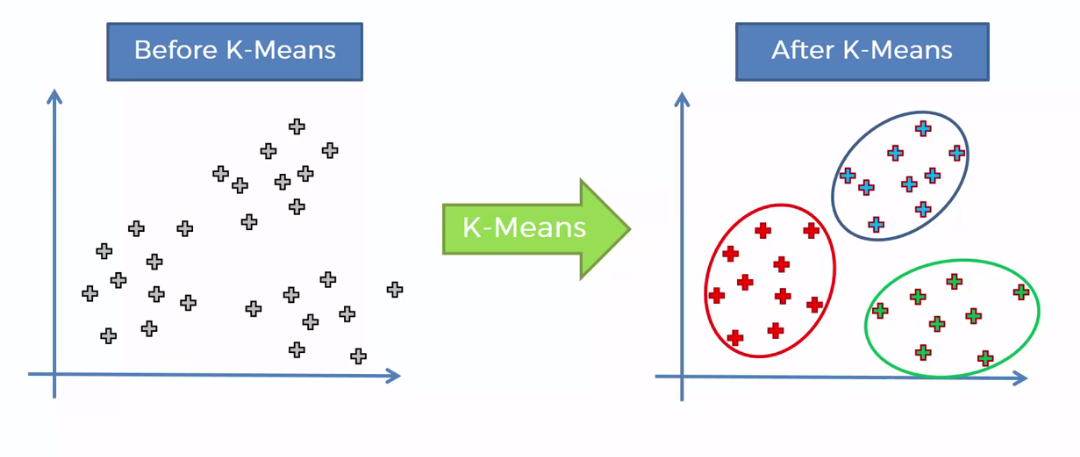• Dimensionality reduction: This refers to representing the same data using lesser dimensions i.e.rows and columns while maintaining its integrity. This is usually used while removing noisy data from an image to improve its quality.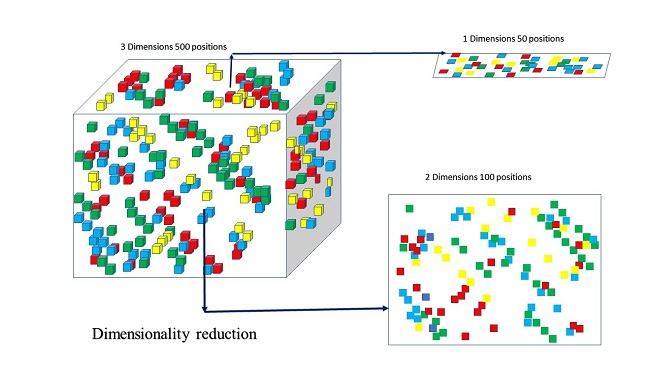## Examples of Unsupervised learning:

• K-means clustering is used to group books into different genres based on their summary. If the summary contains the words "night", "haunted" and "ghost" , then the book is most likely to be of the horror genre.
• In neuroscience, maximally informative dimensions is a dimensionality reduction technique used to project neural responses onto a lower dimensional subspace, without much data loss.
• Clustering is used in recommender systems to group users with similar interests together in order to recommend similar content.
• Businesses use clustering for customer segmentation. In layman's terms, it is understanding different groups of customers so that effective marketing strategies could be built. Below image shows segmentation of customers based on their income and age.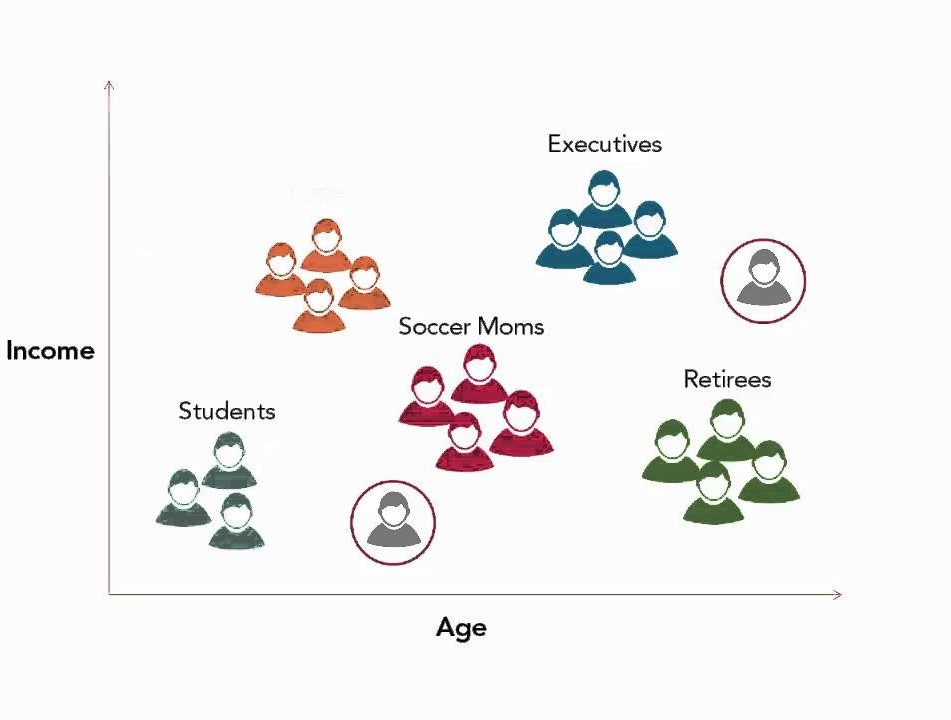• While searching for something in a search engine, we come across similar results showing in suggestions which are a combination of similar matches to our original query. This is also due to clustering and we get suggestions from data across clusters that are more closely related to the data in our query.

# Semi-Supervised learning

Semi-supervised learning falls in-between supervised and unsupervised learning. Here, while training the model, the training dataset comprises of a small amount of labeled data and a large amount of unlabeled data. This can also be taken as an example for weak supervision.

## Examples of Semi-Supervised learning

• An ideal example for the use case of semi-supervised learning is a text document classifier. Since it is nearly impossible to find a large amount of text documents which are labeled, using semi-supervised learning, the model can learn from a small amount of labeled text documents while still classifying a larger amount of unknown text documents.
• Another use case is the classification of contents in the Internet. Labeling and indexing each webpage is an impossible task. So, semi-supervised learning algorithm can be used here so that with just a small amount of labeled webpages, all of them could be indexed and labeled. Even Google uses a variant of this machine learning technique to rank the relevance of a webpage to a given query. As a result, the webpage with highest relevance is displayed first.

# Summary

After understanding about the various types of machine learning techniques, we can summarize them as follows:

### Supervised Learning

• Input data is labeled.
• Used for prediction and classification models.
• It takes direct feedback of the output.
• These models predict the output.
• It can be used in cases where we know the input and their respective outputs.

### Semi-Supervised learning

• A large amount of input data is unlabeled while a small amount is labeled.
• It is used when labeling the whole dataset is expensive.
• It is a type of weak supervision.

### Unsupervised learning

• Input data is unlabeled.
• Used for extracting information from large amounts of data.
• Does not have a feedback mechanism.
• These models find underlying patterns in data.
• We can use these models where we only know the input data and do not have the knowledge of the corresponding output.

With this article at OpenGenus, you must have the complete idea of Supervised, Unsupervised and Semi-Supervised Learning.#### Sanjana Babu

Sanjana Babu is a Student at SRM Valliammai Engineering College and is an Intern at OpenGenus. She is a Philomath.

Supervised, Unsupervised and Semi-Supervised Learning